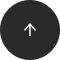Standard SQL

# How to Multiply Two Columns in SQL

#### Operators:

Multiply two columns, JOIN, alias

#### Problem:

want to multiply values from two columns of a table.

#### Example:

Our database has a table named `purchase` with data in the following columns: `id`, `name`, `price`, `quantity`, and `discount_id`.

idnamepricequantitydiscount_id
1pen731
2notebook582
3rubber1131
4pencil case2423

Let’s multiply the price by the quantity of the products to find out how much you paid for each item in your order.

#### Solution:

```SELECT name,
price*quantity  AS total_price
FROM purchase;
```

This query returns records with the name of the product and its total price:

nametotal_price
pen21
notebook40
rubber33
pencil case48

#### Discussion:

Do you need to select the name of each record (in our case, `name`) and compute for it the result of multiplying one numeric column by another (`price` and `quantity`)? All you need to do is use the multiplication operator (*) between the two multiplicand columns (`price` * `quantity`) in a simple `SELECT` query. You can give this result an alias with the `AS` keyword; in our example, we gave the multiplication column an alias of `total_price`.

Note that you can also use data from two columns coming from different tables. We have another table in our database named `discount` that has columns named `id` and `value`; the latter represents the percent discount on the item with the given ID.

idvalue
110
220
330

Look at the example below.

#### Solution:

```SELECT p.name,
p.price*p.quantity*(100-d.value)/100  AS total_price
FROM purchase p
JOIN discount d ON d.id=p.discount_id;
```

Here’s the result:

nametotal_price
pen18.90
notebook32.00
rubber29.70
pencil case33.60

As you can see, it’s quite easy to multiply values from different joined tables. In our example above, we multiplied the price of each product by its quantity from one table (`purchase`) and then multiplied this total price by the percent discount using the `discount` table.

#### Recommended courses: